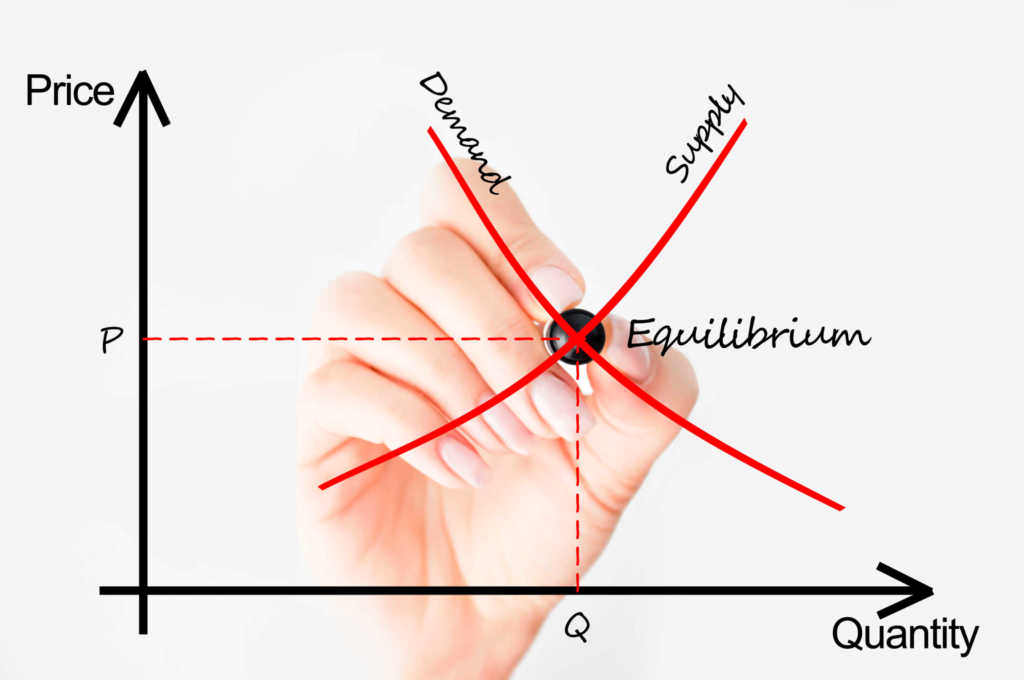# Economics Interview Questions

Below are real examples of the most common macro economic interview questions use to hire in corporate finance.

## What are common economics interview questions?

In finance interviews, it’s common to be asked questions on a wide range of related topics, including being asked a few economics interview questions.

Below is a list of some example questions to give you an idea of what to expect.  While there are an unlimited number of economics questions you could be asked, these questions will give you a sense of the types of questions you could get.### List of economics interview questions

1. What are the correlations among these four asset classes: gold, oil, U.S. 10-year treasuries, and the S&P?
2.  Explain quantitative easing.
3.  Tell me about the shape of the yield curve.
4.  If the U.S. defaults on it’s debt, explain to me what will happen in all the major markets (stocks, bonds, commodities, FX, etc.) and why.
5.  Explain the Yield Curve and its significance.
6. Explain the difference between a regular good and a Veblen good?
7.  What economic indicators are considered by the Federal Reserve when making interest rate decisions?
8.  What is your outlook on the US dollar?
9. Please explain your take on the current macroeconomic situation in China/BRIIC/USA?
10. How do you value a currency?
11.  How would you calculate Value at Risk (VaR)?
12. What’s wrong with VaR as a measurement of risk?
13.  What does it mean for risk when the yield curve is inverted?
14.  How would you hedge against a particular equity/bond under current market conditions?
15. When can hedging an options position mean that you take on more risk?
16.  What are the risks inherent in an interest rate swap?
17. How is inflation adjusted for in trend analysis to make different years comparable?
18. Explain income demand elasticity to me.
19. What is the multiplier effect and how is it related to the GDP of a country?
20. Please explain the difference between causation and correlation.
21. What is an example of a perfectly inelastic good, and why is it such?
22. Explain the difference between liquidity risk and maturity risk.
23. Explain the difference between the nominal risk-free rate and the real risk-free rate.

### More resources for economics interview questions

To prepare answers for the above questions, it’s important to have a solid understanding of the main economic principles and concepts you’ll require.  Reading the The  Economist (https://www.economist.com/) is a great way to stay on top of recent political and economic events.

To start laying the foundation of having a solid understanding of economic principles, explore CFI’s resources library of relevant resources: